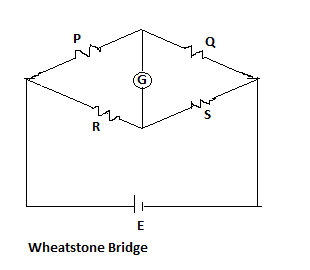# Wheatstone bridge - Balanced equation

Q.  The given figure shows the Wheatstone bridge method for measurement of unknown resistance (R).The balanced equation for Wheatstone bridge is given by
- Published on 05 Nov 15

a. P/R = Q/S
b. P/S = Q/R
c. P/R = S/Q
d. R/P = Q/S